# Divisibility - 5th grade (10y) - math problems

#### Number of problems found: 21

• Primes 2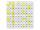Which prime numbers is number 2025 divisible?
• Multiples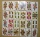What is the sum of the multiples of number 7 that are greater than 30 but less than 56?
• NumberDetermine unknown number if you know that difference between five times and triple of number is 42.
• Sum of the digitsHow many are two-digit natural numbers that have the sum of the digits 9?
• DivisibilityIs the number 761082 exactly divisible by 9? (the result is the integer and/or remainder is zero)
• CherriesCherries in the bowl can be divided equally among 19 or 13 or 28 children. How many are the minimum cherries in the bowl?
• Twos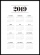Vojta started writing the number of this year 2019202020192020 into the workbook. .. And so he kept going. When he wrote 2020 digits, no longer enjoyed it. How many twos did he write?
• Exhibition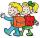The teacher paid for 280 Kč for 4.A students for admission to the exhibition. How many students were on the exhibition?
• LCMCommon multiple of three numbers is 3276. One number is in this number 63 times, second 7 times, third 9 times. What are the numbers?
• DozenWhat is the product of 26 and 5? Write the answer in Arabic numeral. Add up the digits. How many of this is in a dozen? Divide #114 by this
• Class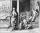When Pythagoras asked how many students attend the school, said: "Half of the students studying mathematics, 1/4 music, seventh silent and there are three girls at school." How many students had Pythagoras at school?
• Perpetrator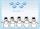The perpetrator is a number that is smaller than the number 80. It is a multiple of five and it is odd. If we added up the tens and ones of this number we get number 8.
• Fluid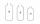We have vessels containing 7 liters, 5 liters and 2 liters. Largest container is filled with fluid the others empty. Can you only by pouring get 5 liters and two 1 liter of fluid? How many pouring is needed?
• Birthdate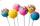Jane on birthday brought 30 lollipops and 24 chewing gum for their friends. How many friends has, if everyone receives the same number of lollipops and chewing gums? How much chewing gum and lollipops got any friend?
• Czech crownsOldrich has one crown. Peter has five crowns coin, a two crown coin and a one-crown coin. Radek has twenty-crown banknote, ten banknote and five-crown coin. The boys got one fifty-one crown and one crown coin. How can they share the money fairly when they
• Buses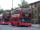At the bus stop is at 10 o'clock met buses No. 2 and No. 9. Bus number 2 runs at an interval of 4 minutes and the bus number 9 at intervals of 9 minutes. How many times the bus meet to 18:00 local time?
• Clotheslines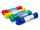Mommy needs 6 clothesline 360 cm long. Clotheslines is sold in the length of eight meters. How many of these clotheslines must buy mom if any does not want to be connected? How much mommy pay for the clotheslines when 1 piece costs 24kč?
• GroupA group of kids wanted to ride. When the children were divided into groups of 3 children, one remain. When divided into groups of 4 children, 1 remains. When divided into groups of 6 children, 1 remains. After divided into groups of 5 children, no one lef
• Czech crowns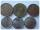Small Petra save money. Grandfather give her 10 crowns. Petra asked his older sister let her calculate how much he has saved up. Sister told her that he has less than 30 crowns. If the sum is divided by three left one crown. If the sum is divided by the f
• Daughters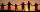The man conducting the census asks a woman to age of three daughters. Woman says when multiply the age getnumber 72; if their ages add up, get a number of our house, as you see. The man says: That is not enough to calculate their ages. She says: my oldest

Do you have an interesting mathematical word problem that you can't solve it? Submit a math problem, and we can try to solve it.

We will send a solution to your e-mail address. Solved examples are also published here. Please enter the e-mail correctly and check whether you don't have a full mailbox.

Please do not submit problems from current active competitions such as Mathematical Olympiad, correspondence seminars etc...

Divisibility - math problems. Examples for 5th grade (the fifth graders).